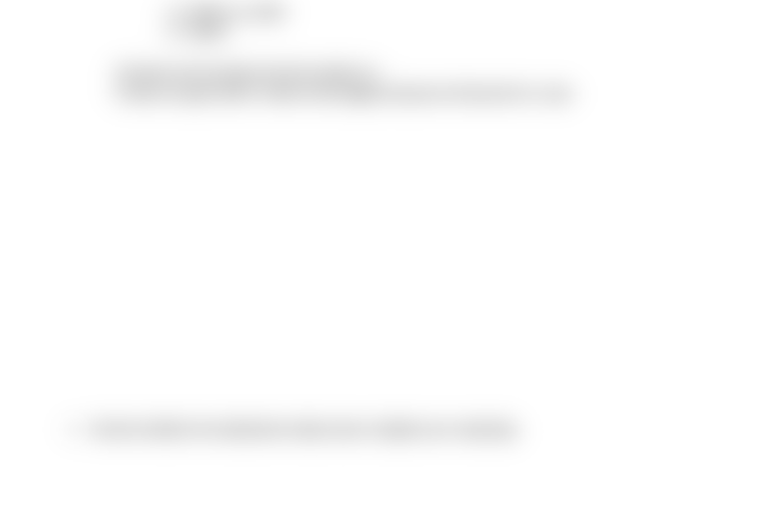Study Guides (400,000)
US (230,000)
FIU (700)
MGF (4)
Final

# MGF 1107 Lecture 4: Conceptual HW 4B JoniHaxhiExam

Department
Mathematics - General and Fini
Course Code
MGF 1107
Professor
Juan
Study Guide
Final

This preview shows half of the first page. to view the full 2 pages of the document.Conceptual Homework 4B
1. Which is the better choice: \$2500 deposited for a year: You must show your work below.
a. at a rate of 6.2% compounded quarterly?
b. at a rate of 5.2% compounded daily?
c. At a rate 4.2% compounded continuously?
a. A = P (1 + APR/n) ^ nY
A = 2500\$ (1 + 0.062/4) ^ 4 x 1
= 2500\$ x 1.0634564532200625
= \$2,658.64113305015625
b. A = 2500\$ (1 + 0.052/365) ^ 365 x 1
A = 2500\$ x 1.0524
A = \$2,631
C. A = P x e ^ (APR x Y)
A = 2500\$ x e ^ (0.042 x 1)
A = 2500\$ x 1.0428
A = \$2607
The best work as shown by the results is a.
In there we get 2.658 \$ which is the highest amount of interest for 1 year.
2. Decide whether the statement makes sense. Explain your reasoning.